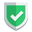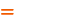我们相信：世界是美好的，你是我也是。平行空间的世界里面，不同版本的生活也在继续...

python代码，如何理解functools类中的wraps和partial函数？从目前的情况来看，`functools`类中的方法，都是一些非常神奇的函数，每个都具有非常神奇的功能。本文中，对其中的`wraps``partial`进行一下了解。

## `wraps`

``````from functools import wraps
def examination2(func):
@wraps(func)
def anyname2(*args, **kwargs):
''' anydoc2 '''
print("准备参加考试2")
func(*args, **kwargs)
print("通过考试拿到证书2")
return anyname2
@examination2
def exam(language="西班牙语"):
''' doc '''
print("参加了考试2"+language)
print(exam.__name__, exam.__doc__)``````

## `partial`

``````import functools

def fn(args1, args2):
if(args1 == ""):
raise TypeError
return [args1, args2]

t = functools.partial(fn)  # 新的函数t
print(t("a1", "a2"))

t2 = functools.partial(fn, "a1")  # 新的函数t2
print(t2("a2"))

t3 = functools.partial(fn, "a1", "a2")  # 新的函数t3
print(t3())``````

`functools.partial`可以传零个参数到所有参数，剩下的参数（如果有）在新的函数里面再次传递。

``````from functools import partial
def fn(args1, args2):
if(args1 == ""):
raise TypeError
return [args1, args2]
t = partial(fn)  # 新的函数t
print(t("a1", "a2"))``````

from functools import partialpartial(fn)
import functoolsfunctools.partial(fn)

## 综述如果本文对您有帮助，或者节约了您的时间，欢迎打赏瓶饮料，建立下友谊关系。本博客不欢迎：各种镜像采集行为。请尊重原创文章内容，转载请保留作者链接。【福利】 腾讯云最新爆款活动！1核2G云服务器首年50元！【源码】本文代码片段及相关软件，请点此获取更多信息【绝密】秘籍文章入口，仅传授于有缘之人python